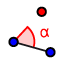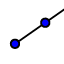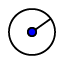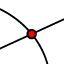# Side-Angle-Side

## SAS Explained

If two sides and the included angle of one triangle are congruent to the corresponding parts of another triangle, the triangles are congruent. The "included angle" in SAS is the angle formed by the two sides of the triangle being used. Below is an example how to construct this. If you change anything in the construction, just click on the arrows on the top right to restore the construction.

## Steps in constructing SAS

Now you try to draw a triangle congruent to the previous one﻿ You need to draw a triangle with side AB=5cm, included angle CAB=35 degrees and side AC=8. Try to do this in the "Applet" below

1. Useto draw segment AB and if you are requested to give the length type in 5
2. Useto draw an angle at point A. (Hint: Always click last on the point where you want the angle.) If requested for the angle size type in 35 degrees. Lastly you need to select clockwise or anti-clockwise. The direction of movement is from the line in a clockwise or anti-clockwise direction.
3. Useto draw a ray from point A through point B' that were created by the angle tool.
4. Useto draw a circle at point A and if requested to enter a radius type in 8
5. Useto place point C at the intersection of the ray and the circle
6. Useto draw triangle ABC